# First Big Data workshop at the Simons Institute

This week at the Simons Institute we had the first Big Data workshop on Succinct Data Representations and Applications. Here I would like to briefly talk about one of the ‘stars’ of this workshop: the squared-length sampling technique. I will illustrate this method on three examples (taken from three seminal papers).

Fast low rank approximation

Frieze, Kannan and Vempala proposed the following algorithm to compute an approximate low rank decomposition of a matrix(this specific version is taken from Chapter 6 here). We denote bythe columns of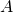.

Letbei.i.d. random variables taking values in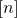whose distribution is proportional to the squared-length of the columns, more precisely the probability that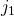is equal tois proportional to. Let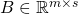be such that itscolumn is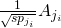. Next compute an SVD ofand letbe the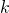top left singular vectors of. The low rank approximation tois finally given byOne can prove that forthis approximation satisfies:where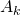is the best rankapproximation to.

The amazing feature of this algorithm is that the complexity of computing the projection matrix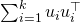is independent of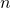(one needs to be careful with how the sampling ofis done but this can be taken care of). In fact it is even possible to obtain an algorithm whose complexity is independent of bothand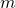and depends only polynomially inand(though the version described above might be better in practice because the complexity is simply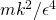).

Fast graph sparsification

Spielman and Teng looked at the following graph sparsification problem: given a weighted graph, find a ‘sparse’ weighted graph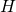such that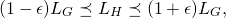where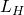andare the graph Laplacians ofand. The idea is that if one is able to find ‘fast enough’ a sparse approximationtothen one can solve many problems on(which is dense) by instead solving them much faster on(think of solving linear systems of the form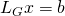for example).

Spielman and Srivastava proposed a method to carry this graph sparsification task using the squared-length sampling technique. First they reduce the problem to the following: given vectors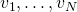that forms a decomposition of the identity, that isfind a small subset of them such that appropriately reweighted they form an approximate decomposition of the identity. Rudelson showed that one can solve this problem by simply sampling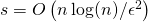vectors fromi.i.d. proportionally to their squared-length and reweight them by the square root of the inverse probability of selecting this vector. In other words, he showed that ifis an i.i.d. sequence insuch that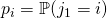is proportional to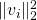then with high probability one hasBatson, Spielman and Srivastava also showed how to find this decomposition with a deterministic algorithm, see also this very nice survey of Naor.

Approximation guarantee for k-means

The last example is the now famous k-means++ algorithm of Arthur and Vassilvitskii. Givenone would like to findminimizing the following quantity:The following strategy gives a randomized-approximation algorithm to this NP-hard problem. First select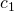at random from. Then select iteratively the new centers at random, by sampling a point at random fromproportionally to their distance squared to the current nearest center.

This entry was posted in Conference/workshop. Bookmark the permalink.

## 2 Responses to "First Big Data workshop at the Simons Institute"

••